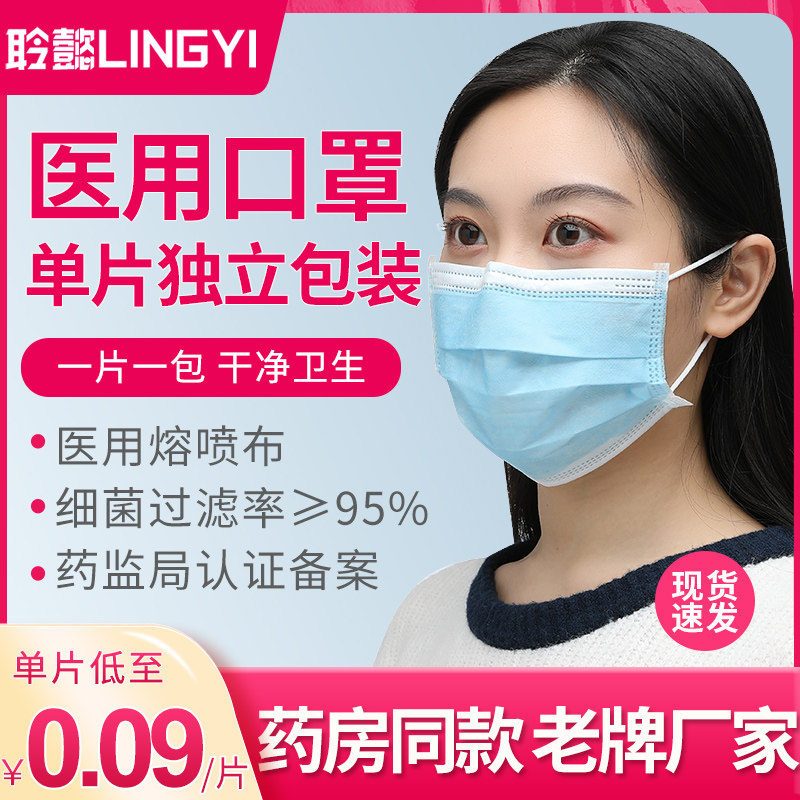# 长下影线选股

【爆款推荐】一次性医用口罩医疗三层防护成人医生专用夏天夏季透气单独立包装 2小时销量达421件 原价9.90元，券后价仅6.90元【立即领券】即可领券购买
【立即下单】

XG:下影线>实体*2 AND 上影线<实体 AND 实体<5 AND C>O;

</o;
</o;

c1:=ref(c,1);
F:=MA(C,5);
F1:=REF(F,1);
c/c1<=1.02 and c1/c<1.02 and c>o and o-l/c-o>=2 AND F>F1;

`我主要想要选的是在底部出现长下影线，因为这时反弹的概率大，这个公式能选出在底部出现的长下影线吗？谢谢`

```c1:=ref(c,1);
F:=MA(C,5);
F1:=REF(F,1);
M:=MA(c,10);
M1:=REF(M,1);

c/c1<=1.02  && (O-L)/(C-O)>=2
&&F>=F1&&COUNT(M=8;

SHITI:=MAX(O,C)-MIN(O,C);
XIAYING:=MIN(O,C)-L;
XIAYING/SHITI>2.5;

SHITI:=MAX(REF(O,1),REF(C,1))-MIN(REF(O,1),REF(C,1));
XIAYING:=MIN(REF(O,1),REF(C,1))-REF(L,1);
SHANGYING:=REF(H,1)-MAX(REF(O,1),REF(C,1));

C1:=REF(C,1);
F:=MA(C,5);
G:=MA(C,20);
F1:=REF(F,1);
F5:=REF(F,5);
F10:=REF(F,10);
F20:=REF(F,20);
F60:=REF(F,60);
G1:=REF(G,1);

XIAYING/SHITI>3 AND SHANGYING/SHITI<2 AND C>F AND F>F1 AND G>=G1;//5日线和20日线都向上抬头，今日收盘价稳在5日线上。

```
```